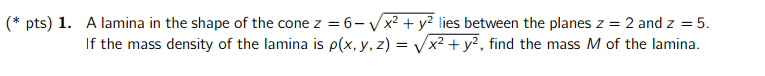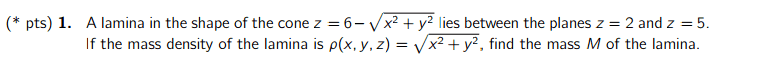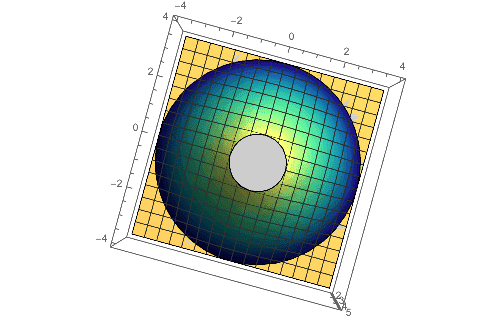# Finding mass from mass density of lamina

## Homework Statement## Homework Equations

m = ∫∫sρ(x,y,z)dS

## The Attempt at a Solution

I used polar coordinates for this (is that necessary here)? I found zx and zy, took the cross product of those, and found it to be √(2). So dS = √2 dA.
√2 02pi25r2drdθ = 78pi√2

Simon Bridge
Homework Helper
Polar coordinates are entirely appropriate: try cylindrical-polar and exploit the symmetry.
Don't know what you mean by ##z_x## and ##z_y## or the role you used ##dS## and ##dA## for.
You have to spell out your reasoning - different courses can use different conventions.

Since it is a lamina - the mass density is probably an area rather than a volume density; so ##dm = \rho\;d\!A## (check).
Here dA is an element of area lying on the surface of the cone and dm comes from: ##m=\int_{Area}\;dm##

To select a strategy you can feel confident with, 1st figure out what ##\rho## depends on.
Does it depend on r? z? ##\theta##? All of them? None of them? Which?
Then a good choice for the geometry of dA may present itself.

Polar coordinates are entirely appropriate: try cylindrical-polar and exploit the symmetry.
Don't know what you mean by ##z_x## and ##z_y## or the role you used ##dS## and ##dA## for.
You have to spell out your reasoning - different courses can use different conventions.

Since it is a lamina - the mass density is probably an area rather than a volume density; so ##dm = \rho\;d\!A## (check).
Here dA is an element of area lying on the surface of the cone and dm comes from: ##m=\int_{Area}\;dm##

To select a strategy you can feel confident with, 1st figure out what ##\rho## depends on.
Does it depend on r? z? ##\theta##? All of them? None of them? Which?
Then a good choice for the geometry of dA may present itself.
Doesn't it depend on all of them?

Polar coordinates are entirely appropriate: try cylindrical-polar and exploit the symmetry.
Don't know what you mean by ##z_x## and ##z_y## or the role you used ##dS## and ##dA## for.
You have to spell out your reasoning - different courses can use different conventions.

Since it is a lamina - the mass density is probably an area rather than a volume density; so ##dm = \rho\;d\!A## (check).
Here dA is an element of area lying on the surface of the cone and dm comes from: ##m=\int_{Area}\;dm##

To select a strategy you can feel confident with, 1st figure out what ##\rho## depends on.
Does it depend on r? z? ##\theta##? All of them? None of them? Which?
Then a good choice for the geometry of dA may present itself.
Okay I'm thinking about dA and would it be dzrdrdθ? The boundaries for z would be from z=2 to z=5, θ would be from 0 to 2pi, but what about r?

Simon Bridge
Homework Helper
Back up a bit.
You have ##\rho(x,y,z)=\sqrt{x^2+y^2}## in post #1.
Notice how that equation does not have a z in it? That means that the value of ##\rho## does not depend on the value of ##z##.
Can you write that equation in terms of the cylindrical-polar coordinates?

Do the same for the equation of the cone.

Back up a bit.
You have ##\rho(x,y,z)=\sqrt{x^2+y^2}## in post #1.
Notice how that equation does not have a z in it? That means that the value of ##\rho## does not depend on the value of ##z##.
Can you write that equation in terms of the cylindrical-polar coordinates?

Do the same for the equation of the cone.
So it only depends on r and θ, correct?
p(x,y,z) = r
z = 6-r

Simon Bridge
Homework Helper
OK - so you have ##\rho = r## ... look at what you wrote: is there a ##\theta## in that equation?

Note: ##\rho(x,y,z)=r## is a bit misleading because x,y,z belong to rectangular coords while the r is cylindrical. You could write ##\rho(r,\theta,z)=r##

OK - so you have ##\rho = r## ... look at what you wrote: is there a ##\theta## in that equation?
No... so it only depends on r?
I am getting confused on what to use now...

Simon Bridge
Homework Helper
You are doing fine. The density only depends on the distance from the z-axis.
This means you could use a shortcut ... you only need dA to be the area of the surface of the cone between r and r+dr.
Technically you could also make dA the area of the cone between z and z+dz (sketch).

If the density depended on the angle as well, then you'd need dA to be the area of the cone between r and r+dr which is also between ##\theta## and ##\theta + d\theta##. You can still do it that way if it helps you think about it.

Last edited:
You are doing fine. The density only depends on the distance from the z-axis.
This means you could use a shortcut ... you only need dA to be the area of the surface of the cone between r and r+dr.

If the density depended on the angle as well, then you'd need dA to be the area of the cone between r and r+dr which is also between ##\theta## and ##\theta + d\theta##. You can still do it that way if it helps you think about it.
I think I see what you are saying... So since this is not dependent on z or theta, would the integrand just be rdr?

Simon Bridge
Homework Helper
No. the integrand is ##\rho\; d\!A##
The area on the cone between r and r+dr is circular so you'd expect to see a ##\pi## in there someplace right?
Did you draw a sketch?

No. the integrand is ##\rho\; d\!A##
The area on the cone between r and r+dr is circular so you'd expect to see a ##\pi## in there someplace right?
Did you draw a sketch?
A cone with its center at (0,0,-6), but between the planes z=2 and z=5, is that correct?

No. the integrand is ##\rho\; d\!A##
The area on the cone between r and r+dr is circular so you'd expect to see a ##\pi## in there someplace right?
Did you draw a sketch?
But pdA = rdr? Actually, would dA = dzrdr?

Can anyone confirm I am thinking correctly? This homework is due tonight. ):

vela
Staff Emeritus
Homework Helper

## Homework Statement## Homework Equations

m = ∫∫sρ(x,y,z)dS

## The Attempt at a Solution

I used polar coordinates for this (is that necessary here)? I found zx and zy, took the cross product of those, and found it to be √(2). So dS = √2 dA.
If I understand what you did, you found ##dS = \sqrt{2}\,dx\,dy## since you differentiated with respect to ##x## and ##y##. Now if you want to use polar coordinates, you need to find the equivalent of ##dx\,dy## in terms of polar coordinates. Do you know what that is?

The other thing you need to do is figure out the bounds of integral. I've attached a top-down view of the lamina, so you can see its projection onto the xy-plane.